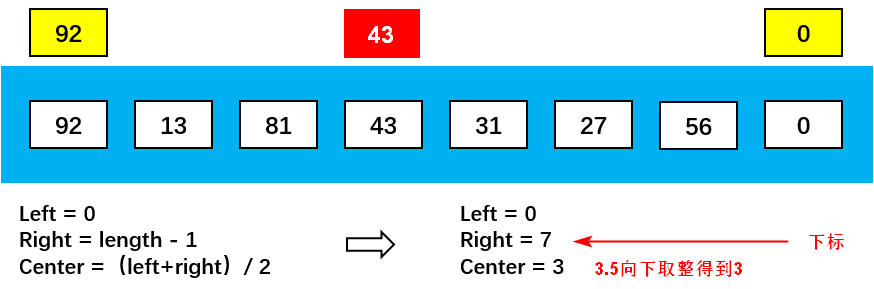# # 快速排序

TIP

## # 算法描述

• 步骤 1：从数列中挑出一个元素，称为 “基准”、“枢纽” （pivot）
• 步骤 2：重新排序数列，所有元素比基准值小的摆放在基准前面，所有元素比基准值大的摆在基准的后面（相同的数可以到任一边）。在这个分区退出之后，该基准就处于数列的中间位置。这个称为分区（partition）操作
• 步骤 3：递归地（recursive）把小于基准值元素的子数列和大于基准值元素的子数列排序

## # 静图演示## # 动图演示## # 选取枢纽

### # 选取方案

• 第一种方案：直接选择第一个元素作为枢纽。但是，当第一个元素就是最小值的情况下，效率不高
• 第二种方案：使用随机数。随机数本身十分消耗性能，不推荐
• 优秀的解决方法
1. 取 index 为头、中、位的三个数据排序后的中位数
2. 当（length-1）/2 不整除时可向下或向上取整### # 代码编写

``````// 交换两个位置的数据：用个临时变量中转一次
function swap(arr, m, n) {
var tmp = arr[m]
arr[m] = arr[n]
arr[n] = tmp
}

// 选择枢纽
function median(arr, left, right) {
// 1.求出中间的位置
var center = Math.floor((left + right) / 2)

// 2.判断大小并且进行交换
if (arr[left] > arr[center]) {
swap(arr, left, center)
}
if (arr[center] > arr[right]) {
swap(arr, center, right)
}
if (arr[left] > arr[center]) {
swap(arr, left, center)
}

// 3.巧妙的操作: 将center移动到right - 1的位置.
swap(arr, center, right - 1)

// 4.返回pivot
return arr[right - 1]
}
``````

### # 代码测试

``````var arr = [3, 6, 4, 2, 11, 10, 5]
var pivot = median(arr, 0, 6)
console.log(pivot) // 3
console.log(arr) // [2, 6, 4, 10, 11, 3, 5]
``````

## # 快速排序

### # 递归代码 1

``````// 快速排序
function quickSort(arr) {
quick(arr, 0, arr.length - 1)
return arr
}
// 递归函数
function quick(arr, left, right) {
// 1. 递归结束条件
if (left >= right) return

// 2. 获取枢纽
var pivot = median(arr, left, right)

// 3. 定义变量，用于记录当前找到的位置
var i = left
var j = right - 1

// 4. 开始进行交换
while (true) {
while (arr[++i] < pivot) {}
while (arr[--j] > pivot) {}

if (i < j) {
swap(arr, i, j)
} else {
break
}
}

// 5. 将枢纽放到正确的位置，i的位置
swap(arr, i, right - 1)

// 6. 分而治之
quick(arr, left, i - 1)
quick(arr, i + 1, right)
}
``````

### # 代码说明

• 第一步：获取枢纽
• 第二步：定义两个指针，从前和后两端分别开始往中间移动，不停地交换位置，将数组分成两组
• 第三步：分别针对 左右两组进行递归 1~2 步

### # 代码测试

``````// 测试快速排序
var arr = [3, 6, 4, 2, 11, 10, 5]
console.log(quickSort(arr).join("-"))
// 2-3-4-5-6-10-11
``````

## # 排序效率

• 快速排序最坏情况下的效率：
1. 每次选择的枢纽都是最左边或最右边的数据，此时效率等同于冒泡排序，时间复杂度为 O(N²)
2. 一般会采取取中位数的方式来避免
• 快速排序的平均效率：为 O(N*logN)
• 虽然其他算法效率也可达到 O(N*logN)，但是其中快速排序是最好的

## # 完整代码

``````/* 快速排序 */

// 交换两个位置的数据：用个临时变量中转一次
function swap(arr, m, n) {
var tmp = arr[m]
arr[m] = arr[n]
arr[n] = tmp
}

// 选择枢纽
function median(arr, left, right) {
// 1.求出中间的位置
var center = Math.floor((left + right) / 2)

// 2.判断大小并且进行交换
if (arr[left] > arr[center]) {
swap(arr, left, center)
}
if (arr[center] > arr[right]) {
swap(arr, center, right)
}
if (arr[left] > arr[center]) {
swap(arr, left, center)
}

// 3.巧妙的操作: 将center移动到right - 1的位置.
swap(arr, center, right - 1)

// 4.返回pivot
return arr[right - 1]
}

// 快速排序
function quickSort(arr) {
quick(arr, 0, arr.length - 1)
return arr
}
// 递归函数
function quick(arr, left, right) {
// 1. 递归结束条件
if (left >= right) return

// 2. 获取枢纽
var pivot = median(arr, left, right)

// 3. 定义变量，用于记录当前找到的位置
var i = left
var j = right - 1

// 4. 开始进行交换
while (true) {
while (arr[++i] < pivot) {}
while (arr[--j] > pivot) {}

if (i < j) {
swap(arr, i, j)
} else {
break
}
}

// 5. 将枢纽放到正确的位置，i的位置
swap(arr, i, right - 1)

// 6. 分而治之
quick(arr, left, i - 1)
quick(arr, i + 1, right)
}

// 测试中位数选取
var arr = [3, 6, 4, 2, 11, 10, 5]
var pivot = median(arr, 0, 6)
console.log(pivot) // 3
console.log(arr) // [2, 6, 4, 10, 11, 3, 5]

// 测试快速排序
var arr = [3, 6, 4, 2, 11, 10, 5]
console.log(quickSort(arr).join("-"))
// 2-3-4-5-6-10-11
``````